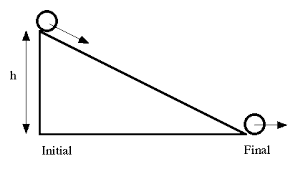# Ball rolling down incline

• df102015

## Homework StatementThe image above shows a basketball (a thin spherical shell I=⅔ mR^2) rolling don an incline of height 8.4 m. If the ball is already rolling with an initial linear speed of 3.0 m/s then what will be the final linear speed when it rolls off the incline?

## Homework Equations

I = 2/3 m R^2
KE = 1/2 m v^2 + 1/2 I ω^2
ω = v / R
PE = m g h

## The Attempt at a Solution

I know that i am missing some equations but honestly i don't know where to start other than substituting for ω and I.

KE = 1/2 m v^2 + 1/2 (2/3 m R^2) [(v / R)^2]

i do not think that i need the potential energy equation, but i mentioned it just incase. Also, how do i cancel out the mass and radius since they are not given in the problem?

You have all the equations. You just need the concept. Is anything conserved in this problem?

•df102015
You have all the equations. You just need the concept. Is anything conserved in this problem?
Energy, so would i set KE=PE ?

Energy, yes. But there is no law that says that KE should always equal PE. What about total mechanical energy: E = KE + PE? What can you say about E as the ball rolls down the slope?

•df102015
Hint:
Total Initial Energy = Total final energy
(As the ball rolls without slipping no heat is lost due to friction)

•df102015
Okay so would i use the equation that i have already set up? ...
KE = 1/2 m v^2 + 1/2 (2/3 m R^2) [(v / R)^2]
And would i add PE (mgh) and set equal to ME?

Hint:
Total Initial Energy = Total final energy
(As the ball rolls without slipping no heat is lost due to friction)
But how do i set the equations up so that the variables i need cancel and everything else remains?

But how do i set the equations up so that the variables i need cancel and everything else remains?
Do what you proposed in post #6 (add in PE), write the KE+PE expressions for each of the starting and ending circumstances, and set them equal.

•df102015
Do what you proposed in post #6 (add in PE), write the KE+PE expressions for each of the starting and ending circumstances, and set them equal.
Okay i think i have it...
1/2 m Vinitial^2 + 1/2 (2/3 m R^2) [(Vinitial / R)^2] + m g h = 1/2 m Vfinal^2 + 1/2 (2/3 m R^2) [(Vfinal / R)^2]

Is this correct?

Okay i think i have it...
1/2 m Vinitial^2 + 1/2 (2/3 m R^2) [(Vinitial / R)^2] + m g h = 1/2 m Vfinal^2 + 1/2 (2/3 m R^2) [(Vfinal / R)^2]

Is this correct?
Yes.

•df102015
Yes.
Thanks! I got the right answer!